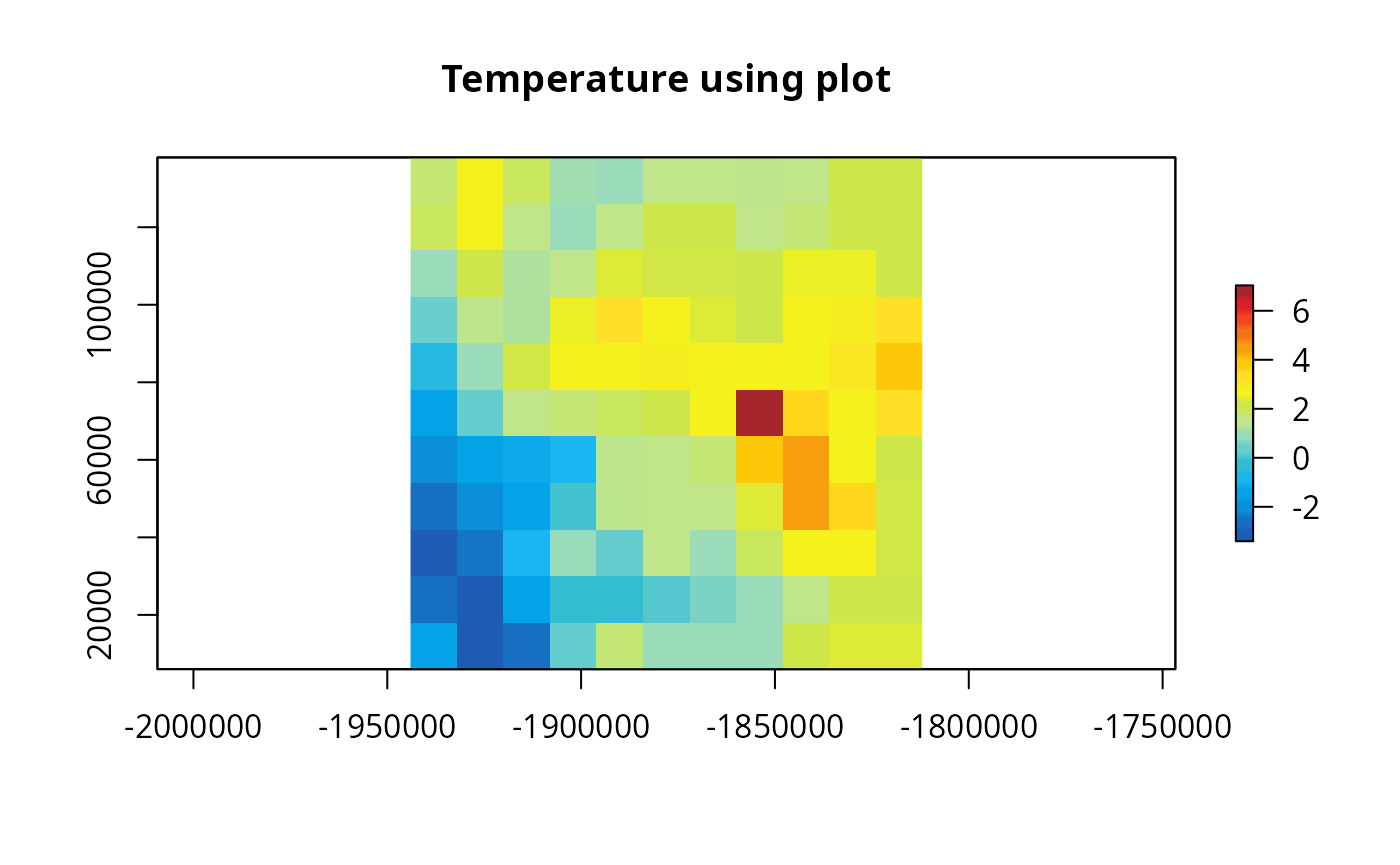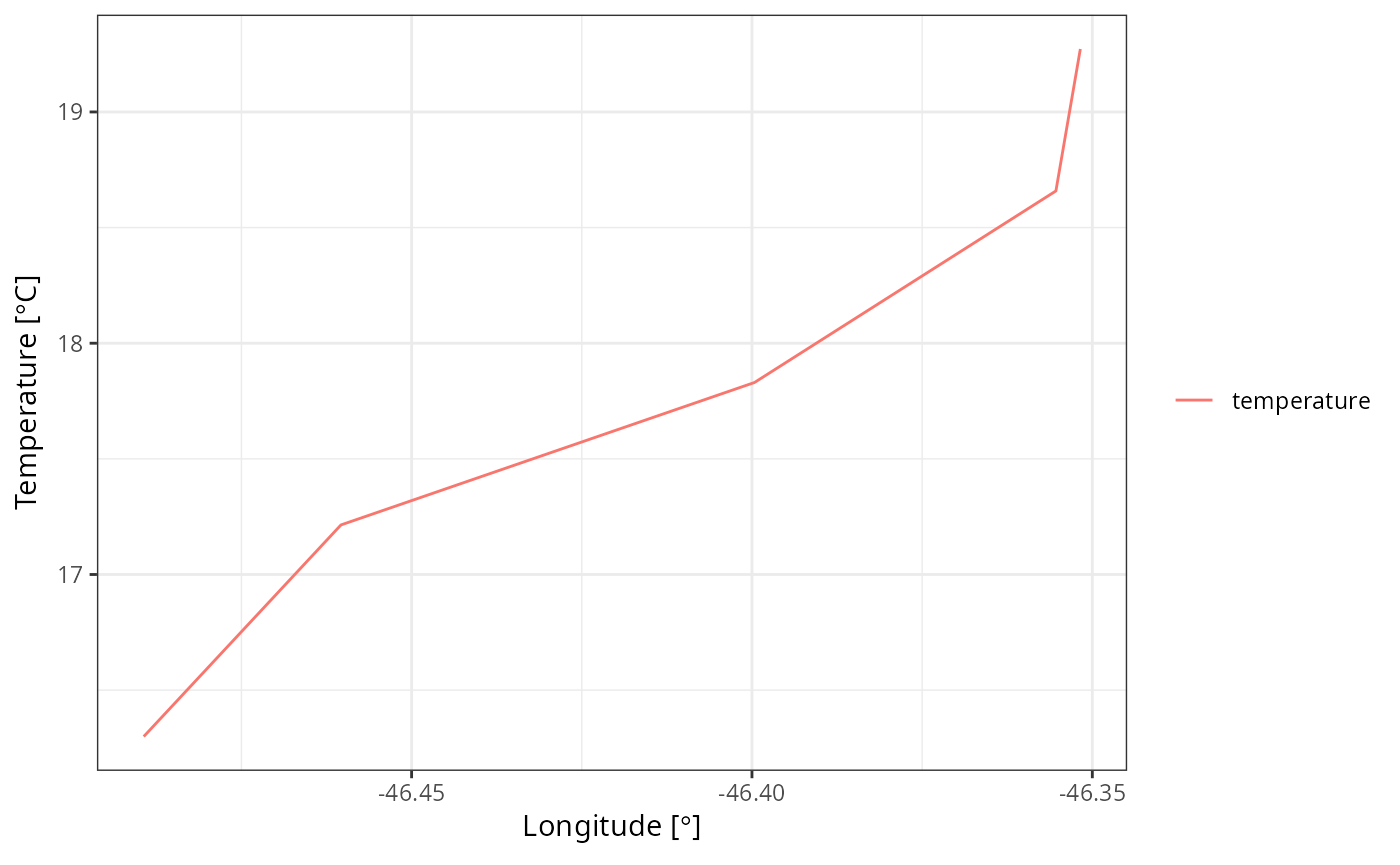Based on this NCL

library(eixport)
#> The legacy packages maptools, rgdal, and rgeos, underpinning the sp package,
#> which was just loaded, will retire in October 2023.
#> Please refer to R-spatial evolution reports for details, especially
#> https://r-spatial.org/r/2023/05/15/evolution4.html.
#> It may be desirable to make the sf package available;
#> package maintainers should consider adding sf to Suggests:.
#> The sp package is now running under evolution status 2
#>      (status 2 uses the sf package in place of rgdal)
library(raster)
library(stars)
#> Linking to GEOS 3.11.1, GDAL 3.6.2, PROJ 9.1.1; sf_use_s2() is TRUE
library(cptcity)
library(sf)
library(vein)
library(ggplot2)

Reading Temperature and crop coast lines for our study area

wrfo <- "/media/sergio/ext5/WRF4/WRF/test/em_real/wrfout_d01_2014-10-03_00:00:00"

t2 <- wrf_get(wrfo, "T2", as_raster = T)
t2 <- t2 -273.15

Find colour palette for temperature

find_cpt("temperature")
#>  "arendal_temperature"    "idv_temperature"        "jjg_misc_temperature"
#>  "kst_03_red_temperature"

Let us create a line between c(-46.5,-23.85) and c(-46.35, -23.95)

m <- cbind(c(-46.5, -46.35),  # xini xend
c(-23.85, -23.95)) # yini yend
cross = st_linestring(m)
(cross <- st_sfc(cross, crs = 4326))
#> Geometry set for 1 feature
#> Geometry type: LINESTRING
#> Dimension:     XY
#> Bounding box:  xmin: -46.5 ymin: -23.95 xmax: -46.35 ymax: -23.85
#> Geodetic CRS:  WGS 84
#> LINESTRING (-46.5 -23.85, -46.35 -23.95)
plot(t2$T2, main = "Temperature using plot", col = cpt("arendal_temperature")) plot(cross, add = T)Now, define several lines m2 <- cbind(c(-46.05, -46.36), # xini xend c(-23.85, -23.95)) # yini yend Define a helper function points_extract <- function(m, sta) { cross = st_linestring(m) cross <- st_sfc(cross, crs = 4326) t2s <- st_as_sf(sta) lt <- st_intersection(t2s, cross) geo <- st_geometry(lt) lt <- st_set_geometry(lt, NULL) na <- names(lt) lt$id <- 1:nrow(lt)
dx <- vein::wide_to_long(df = lt,
column_with_data = na,
column_fixed = "id")
stf <- st_sf(dx, geometry = geo)
lt <- st_centroid(stf)
lt <- cbind(lt, st_coordinates(lt))

return(lt)
}
sta = st_as_stars(t2)
sta <- st_transform(sta, 4326)
names(sta) <- "temperature"
df <- points_extract(m, sta = sta)
#> Warning: attribute variables are assumed to be spatially constant throughout
#> all geometries
#> Warning: st_centroid assumes attributes are constant over geometries

Let us check the data

head(df)
#> Simple feature collection with 5 features and 5 fields
#> Geometry type: POINT
#> Dimension:     XY
#> Bounding box:  xmin: -46.48935 ymin: -23.94883 xmax: -46.35176 ymax: -23.85711
#> Geodetic CRS:  WGS 84
#>         V1 V2          V3         X         Y                    geometry
#> 1 16.29934  1 temperature -46.48935 -23.85711 POINT (-46.48935 -23.85711)
#> 2 17.21432  2 temperature -46.46038 -23.87644 POINT (-46.46038 -23.87644)
#> 3 17.83019  3 temperature -46.39963 -23.91695 POINT (-46.39963 -23.91695)
#> 4 18.65838  4 temperature -46.35535 -23.94644 POINT (-46.35535 -23.94644)
#> 5 19.27224  5 temperature -46.35176 -23.94883 POINT (-46.35176 -23.94883)

Add time variable, select and plot

ggplot(df,
aes(x = X, y = V1, colour = V3)) +
labs(y =expression(paste("Temperature [",degree,"C]")),
x = expression(paste("Longitude [",degree,"]")))+
geom_line() +
theme_bw()+
theme(legend.title = element_blank())